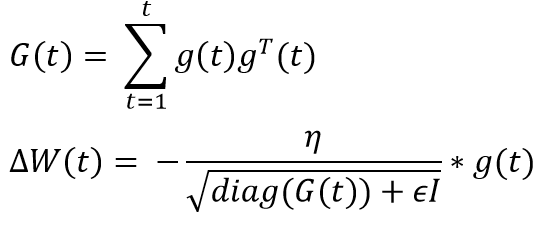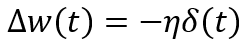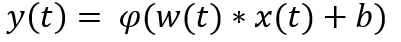Adaptive Learning Rate methodologies like AdaGrad and RMSprop, we let these optimizer tune the learning rate by learning the characteristics of the underlying data. These optimizers give frequently occurring features low learning rates and infrequent features high learning rates thus converging faster.

In my earlier post Gradient Descent with Momentum, we saw how learning rate(η) affects the convergence. Setting the learning rate too high can cause oscillations around minima and setting it too low, slows the convergence. Learning Rate(η) in Gradient Descent and its variations like Momentum is a hyper-parameter which needs to be tuned manually for all the features.Image by author

When we use the above equation for updating weights in a neural net

1. Learning rate is the same for all the features
2. Learning rate is the same at all the places in the cost space

## Impact of constant learning rate on the convergence

Suppose, we are trying to predict the success/rating of a movie. Let's assume there are thousands of features and one of them being “is_director_nolan”. The feature “is_director_nolan” in our input space will be mostly 0 as Nolan has directed very few movies but his presence significantly impacts the success/rating of a movie. Essentially, this feature will be sparse but because of high information content, we cannot ignore it.

During the forward pass in a neural net, if the input(x)at iteration(t) is 0 then the output becomes the activation(φ) of bias(b) using the below equation.Image by author

Hence, the local gradient calculated during back prop w.r.t this constant bias will be 1 and the weight update will be very small for this feature (look at the first equation)

For a sparse input feature like “is_director_nolan”, a large weight update will happen only when the input changes from 0 to 1 or vice versa. Whereas a dense feature will receive more updates. Therefore, using a constant and same learning rate for all the features is not a good idea.

## HTML entity encoder decoder Online

Ever wondered how the machine learning algorithms give us the optimal result, whether it is prediction, classification or any other? How…

## Learning Rates and the Convergence of Gradient Descent

In the previous article we dived into the fundamental theory behind standard backpropagation and also introduced the different aspects that are responsible for practically optimizing the process.

## What is Supervised Machine Learning

What is neuron analysis of a machine? Learn machine learning by designing Robotics algorithm. Click here for best machine learning course models with AI

## Pros and Cons of Machine Learning Language

AI, Machine learning, as its title defines, is involved as a process to make the machine operate a task automatically to know more join CETPA

## How To Get Started With Machine Learning With The Right Mindset

You got intrigued by the machine learning world and wanted to get started as soon as possible, read all the articles, watched all the videos, but still isn’t sure about where to start, welcome to the club.